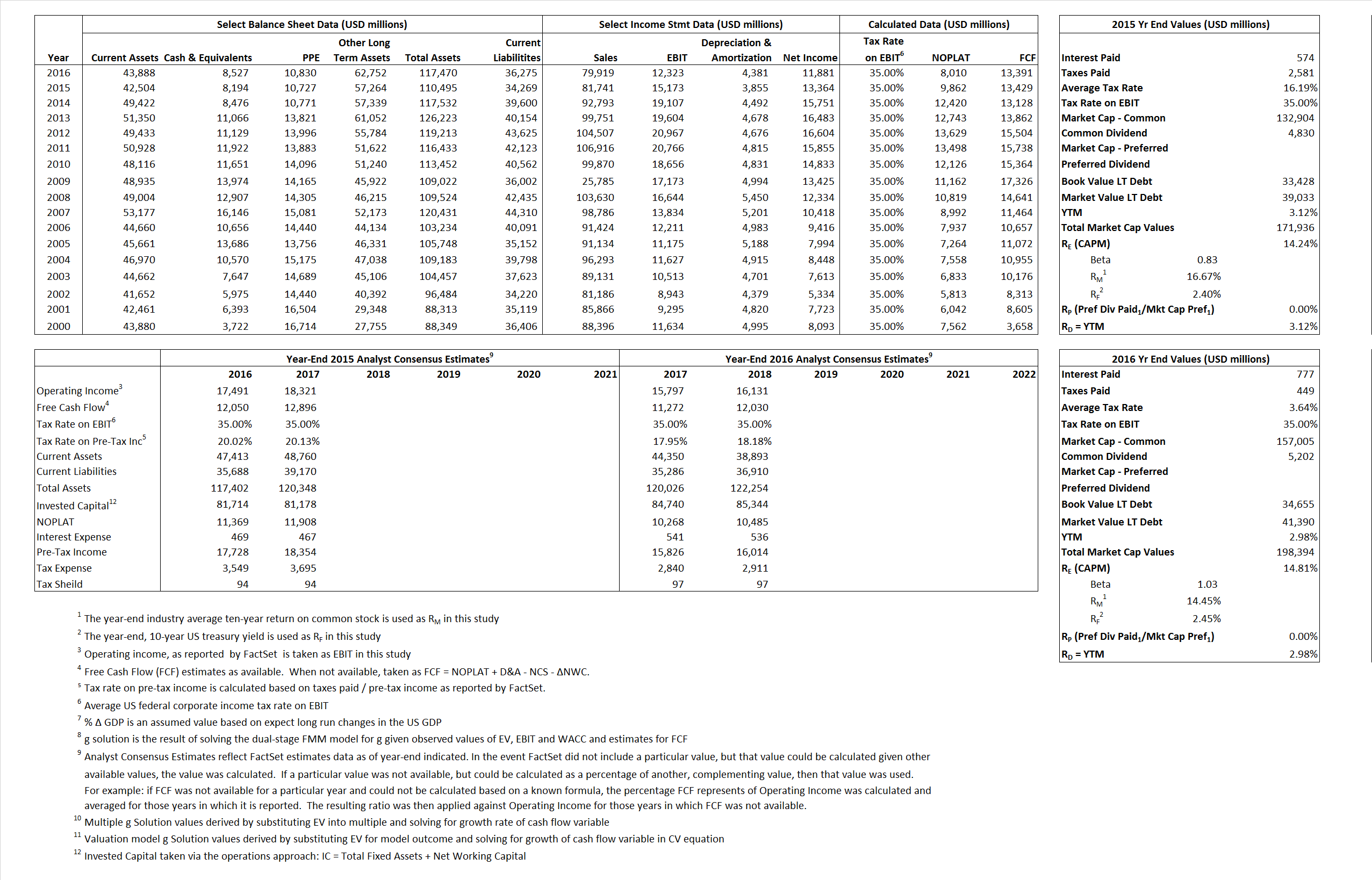## Analyst Listing

The following analysts provide coverage for the subject firm as of May 2016:

 Broker Analyst Analyst Email RBC Capital Markets Amit Daryanani amit.daryanani@rbccm.com Drexel Hamilton Brian J. White bwhite@drexelhamilton.com Stifel Nicolaus David Grossman dgrossman@stifel.com JMP Securities Greg Mcdowell gmcdowell@jmpsecurities.com Jefferies James Kisner jkisner@jefferies.com Atlantic Equities Josep Bori j.bori@atlantic-equities.com Cantor Fitzgerald Joseph D. Foresi jforesi@cantor.com Daiwa Securities Co. Ltd. Kazuya Nishimura kazuya.nishimura@us.daiwacm.com BMO Capital Markets Keith Bachman keith.bachman@bmo.com Credit Suisse Kulbinder Garcha kulbinder.garcha@credit-suisse.com Wells Fargo Securities Maynard J. Um maynard.um@wellsfargo.com Societe Generale Richard Nguyen richard.nguyen@sgcib.com Deutsche Bank Research Sherri Scribner sherri.scribner@db.com Bernstein Research Toni M. Sacconaghi Jr sacconaghi@bernstein.com

## Primary Input Data## Derived Input Data

### Equational Form

Net Operating Profit Less Adjusted Taxes NOPLAT  9,862  8,010$NOPLAT\, =\, EBIT\, x\, (1 \,-\, Avg \,\,Tax\,\, Rate\,\, on\,\, EBIT)$
Free Cash Flow FCF  13,429 13,391$FCF\,=NOPLAT\,+\,Non-Cash\,Expenses-\Delta NWC\,-\,NCS$
Tax Shield TS 93 28$TS\,=\,Interest\,\,Paid\,\,x\,\, Avg \,\,Tax\,\,Rate\,\, on\,\, Pre-Tax\,\, Income$
Invested Capital IC  76,226 81,195$IC\,=\,Fixed\,\,Operating\,\,Assets\,\,+\,\,Net\,\, Working\,\, Capital$
Return on Invested Capital ROIC  12.94% 9.87%$ROIC\,=\,\frac { NOPLAT }{ IC }$
Net Investment NetInv 2,149  9,350$NetInv\,=\,{ {IC}_{1}}-{{IC}_{0}}+Depreciation$
Investment Rate IR  21.79% 116.73%$IR\,=\,\frac {NetInv}{NOPLAT}$
Weighted Average Cost of Capital
WACCMarket  11.60%  12.32%$WACC\,=\,\frac { E }{ V } { R }_{ E }\,+\,\frac { P }{ V } { R }_{ P }\,+\,\frac { D }{ V } { R }_{ D }\left( 1- Avg\,\, Tax\,\,Rate\,\,on\,\,Pre-Tax\,\,Income \right)$
WACCBook   6.79%   7.77%
Enterprise value
EVMarket  163,742  189,867$EV\,=\,Market\,\,Cap\,\,Equity\,+\,\,Long\,\,Term\,\,Debt\,-\,Cash$
EVBook  158,138  183,133
Long-Run Growth
g = IR x ROIC
2.82%  11.52% Long-run growth rates of the income variable are used in the Continuing Value portion of the valuation models.
g = %$\Delta$ GDP   2.50%   2.50%
Margin from Operations M  18.56%  15.42%$M\,\,=\,\,\frac{EBIT}{SALES}$
Depreciation/Amortization Rate D  20.26%  26.23%$D\,\,=\,\,\frac{D+A}{EBITDA}$

## Valuation Multiple Outcomes

The outcomes presented in this study are the result of original input data, derived data, and synthesized inputs.

### model g solution

12/31/2015 12/31/2016 12/31/2015 12/31/2016 12/31/2015 12/31/2016

EV/SALES$\frac {EV}{Sales} \,= \,\frac{ROIC\, -\, g}{ROIC\,(WACC\,-\,g)}\,(1\,-\,T)\,(M)$

2.00  2.38  10.44%  14.15%  10.60%  13.58%

EV/EBITDA$\frac {EV}{EBITDA} \,= \,\frac{ROIC\, -\, g}{ROIC\,(WACC\,-\,g)}\,(1\,-\,T)\,(1\,-\,D)$

8.61 11.37 10.44% 14.15% 10.60% 13.58%

EV/NOPLAT$\frac {EV}{NOPLAT} \,= \,\frac{ROIC\, -\, g}{ROIC\,(WACC\,-\,g)}$

16.60 23.70 10.44% 14.15% 10.60% 13.58%

EV/FCFOPS$\frac {EV}{FCF_{OPS}} \,= \,\frac{ROIC\, -\, g}{ROIC\,(WACC\,-\,g)}\,(1\,-\,T)$

12.19 14.18 10.44% 14.15% 10.60% 13.58%

EV/EBIT$\frac {EV}{EBIT} \,= \,\frac{ROIC\, -\, g}{ROIC\,(WACC\,-\,g)}\,(1\,-\,T)$

10.79 15.41 10.44% 14.15% 10.60% 13.58%

EV/IC$\frac {EV}{IC} \,= \,\frac{ROIC\, -\, g}{WACC\,-\,g}$

2.15 2.34 10.44% 14.15% 10.60% 13.58%